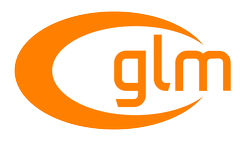0.9.9 API documentation
Vector Relational Functions

Relational and equality operators (<, <=, >, >=, ==, !=) are defined to operate on scalars and produce scalar Boolean results. More...

## Functions

template<length_t L, qualifier Q>
GLM_FUNC_DECL GLM_CONSTEXPR bool all (vec< L, bool, Q > const &v)
Returns true if all components of x are true. More...

template<length_t L, qualifier Q>
GLM_FUNC_DECL GLM_CONSTEXPR bool any (vec< L, bool, Q > const &v)
Returns true if any component of x is true. More...

template<length_t L, typename T , qualifier Q>
GLM_FUNC_DECL GLM_CONSTEXPR vec< L, bool, Q > equal (vec< L, T, Q > const &x, vec< L, T, Q > const &y)
Returns the component-wise comparison of result x == y. More...

template<length_t L, typename T , qualifier Q>
GLM_FUNC_DECL GLM_CONSTEXPR vec< L, bool, Q > greaterThan (vec< L, T, Q > const &x, vec< L, T, Q > const &y)
Returns the component-wise comparison of result x > y. More...

template<length_t L, typename T , qualifier Q>
GLM_FUNC_DECL GLM_CONSTEXPR vec< L, bool, Q > greaterThanEqual (vec< L, T, Q > const &x, vec< L, T, Q > const &y)
Returns the component-wise comparison of result x >= y. More...

template<length_t L, typename T , qualifier Q>
GLM_FUNC_DECL GLM_CONSTEXPR vec< L, bool, Q > lessThan (vec< L, T, Q > const &x, vec< L, T, Q > const &y)
Returns the component-wise comparison result of x < y. More...

template<length_t L, typename T , qualifier Q>
GLM_FUNC_DECL GLM_CONSTEXPR vec< L, bool, Q > lessThanEqual (vec< L, T, Q > const &x, vec< L, T, Q > const &y)
Returns the component-wise comparison of result x <= y. More...

template<length_t L, qualifier Q>
GLM_FUNC_DECL GLM_CONSTEXPR vec< L, bool, Q > not_ (vec< L, bool, Q > const &v)
Returns the component-wise logical complement of x. More...

template<length_t L, typename T , qualifier Q>
GLM_FUNC_DECL GLM_CONSTEXPR vec< L, bool, Q > notEqual (vec< L, T, Q > const &x, vec< L, T, Q > const &y)
Returns the component-wise comparison of result x != y. More...

## Detailed Description

Relational and equality operators (<, <=, >, >=, ==, !=) are defined to operate on scalars and produce scalar Boolean results.

For vector results, use the following built-in functions.

In all cases, the sizes of all the input and return vectors for any particular call must match.

Include <glm/vector_relational.hpp> to use these core features.

GLM_EXT_vector_relational

## Function Documentation

 GLM_FUNC_DECL GLM_CONSTEXPR bool glm::all ( vec< L, bool, Q > const & v )

Returns true if all components of x are true.

Template Parameters
 L An integer between 1 and 4 included that qualify the dimension of the vector.
GLSL all man page
GLSL 4.20.8 specification, section 8.7 Vector Relational Functions
 GLM_FUNC_DECL GLM_CONSTEXPR bool glm::any ( vec< L, bool, Q > const & v )

Returns true if any component of x is true.

Template Parameters
 L An integer between 1 and 4 included that qualify the dimension of the vector.
GLSL any man page
GLSL 4.20.8 specification, section 8.7 Vector Relational Functions
 GLM_FUNC_DECL GLM_CONSTEXPR vec glm::equal ( vec< L, T, Q > const & x, vec< L, T, Q > const & y )

Returns the component-wise comparison of result x == y.

Template Parameters
 L An integer between 1 and 4 included that qualify the dimension of the vector. T A floating-point, integer or bool scalar type.
GLSL equal man page
GLSL 4.20.8 specification, section 8.7 Vector Relational Functions
 GLM_FUNC_DECL GLM_CONSTEXPR vec glm::greaterThan ( vec< L, T, Q > const & x, vec< L, T, Q > const & y )

Returns the component-wise comparison of result x > y.

Template Parameters
 L An integer between 1 and 4 included that qualify the dimension of the vector. T A floating-point or integer scalar type.
GLSL greaterThan man page
GLSL 4.20.8 specification, section 8.7 Vector Relational Functions
 GLM_FUNC_DECL GLM_CONSTEXPR vec glm::greaterThanEqual ( vec< L, T, Q > const & x, vec< L, T, Q > const & y )

Returns the component-wise comparison of result x >= y.

Template Parameters
 L An integer between 1 and 4 included that qualify the dimension of the vector. T A floating-point or integer scalar type.
GLSL greaterThanEqual man page
GLSL 4.20.8 specification, section 8.7 Vector Relational Functions
 GLM_FUNC_DECL GLM_CONSTEXPR vec glm::lessThan ( vec< L, T, Q > const & x, vec< L, T, Q > const & y )

Returns the component-wise comparison result of x < y.

Template Parameters
 L An integer between 1 and 4 included that qualify the dimension of the vector. T A floating-point or integer scalar type.
GLSL lessThan man page
GLSL 4.20.8 specification, section 8.7 Vector Relational Functions
 GLM_FUNC_DECL GLM_CONSTEXPR vec glm::lessThanEqual ( vec< L, T, Q > const & x, vec< L, T, Q > const & y )

Returns the component-wise comparison of result x <= y.

Template Parameters
 L An integer between 1 and 4 included that qualify the dimension of the vector. T A floating-point or integer scalar type.
GLSL lessThanEqual man page
GLSL 4.20.8 specification, section 8.7 Vector Relational Functions
 GLM_FUNC_DECL GLM_CONSTEXPR vec glm::not_ ( vec< L, bool, Q > const & v )

Returns the component-wise logical complement of x.

/!\ Because of language incompatibilities between C++ and GLSL, GLM defines the function not but not_ instead.

Template Parameters
 L An integer between 1 and 4 included that qualify the dimension of the vector.